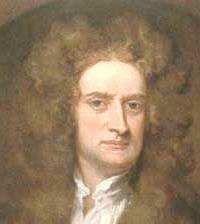## The Most Important Equation in Physics

Almost everyone has heard of the equation E = mc2. And indeed this is the most famous equation in physics, establishing an equivalence between energy and mass. But is this the most important equation in physics? Knowledgeable scientists will tell you no. The most important equation in physics is F = ma, also known as Newton's second law of mechanics. It governs the behavior of everything that is seen and unseen on Earth and in the cosmos -- from the trajectory of a baseball to the motion of a planet.

Students of science and engineering devote half of the time of a classical mechanics course studying and learning how to apply this equation. Thus if you understand F = ma, you know a lot of physics.Sir Isaac Newton (1642-1727)
The Scientist Responsible for the Most Important Equation in Physics*footnote

So what does the equation mean? It can be rewritten in an equivalent and more intuitively understandable form as a = F/m. Cast in this way, Newton's second law provides the response of a body of mass m to a force F. Forces are things that cause changes in motion. Such a change is called acceleration and is denote by the symbol a. A body is undergoing acceleration if it changes its speed or changes its direction of motion.

When you push something, you are inflicting a force upon it. Hence, if you strike a vase with your hand knocking it over, the vase feels a force and undergoes a change of motion. Clearly, a vase lying on its side has undergone a change in state.

Many equations in all kinds of fields have the form

(response) = (driving effect)/(resisting effect)

and Newton's second law is of this structure. Comparing the above to a = F/m, one sees that the resisting effect is the mass of an object, which is sometimes called inertia meaning "the tendency to remain still." Indeed, it is very hard to change the motion of a heavy object. Imagine five people trying to push a car. On the other hand, light objects are easy to accelerate. You don't need five physicists to screw in a light bulb.

In the form F = ma, Newtons' second law tells us the force F needed to implement an acceleration a on a body of mass m. As explained above, force is the driving impetus that causes bodies to accelerate. Examples of forces are gravity, which for example makes things to fall to the ground and causes the planets to move around the Sun; friction, which slows down objects as they rub against another substance; the electric force, which makes charged bodies repel or attract each other and is responsible for the flow of electricity; the magnetic force, which for examples deflects the needle of a compass; buoyancy, which makes things float; and so on. There are countless forces in nature, all creating changes in movement.

Newton's first law of mechanics says that, unless acted upon by forces, a body at rest will remain at rest or a body in motion will remain in motion moving with the same speed and direction. This first law actually follows from the second: If F = 0, then a = F/m = 0 also, and if an object doesn't accelerate then it doesn't change its motion.

A world without forces would be very dull indeed. All bodies at rest would remain at rest; all moving objects would travel at constant speeds in fixed directions forever. There would be no change in movements. All would be predictable but boringly so.

Summarizing, Newton's second law provides a mechanical means for determining the motions of objects. To determine the future movement of a body, one needs to know its mass m, the force F acting on it and its current state of motion. Then one can determine the change in motion about to occur, also known as its current acceleration a, from a = F/m.

This above discussion is an intuitive introduction to the most important equation in physics. If you would like to see some numerical examples, click here.

------------
*footnote Isaac Newton did not explicitly write down the second law in the form F = ma: It was actually Leonhard Euler who expressed it this way.

To the top of this file.

This webpage was prepared by Dr. Stuart Samuel, who has given Jupiter Scientific Publishing permission to use this page and who is the spokesperson for The Bible According to Einstein: A Scientific Complement to the Holy Bible published by Jupiter Scientific, an organization devoted to the promotion of science through books, the internet and other means of communication.

This web page may NOT be copied onto other web sites, but other sites may link to this page.